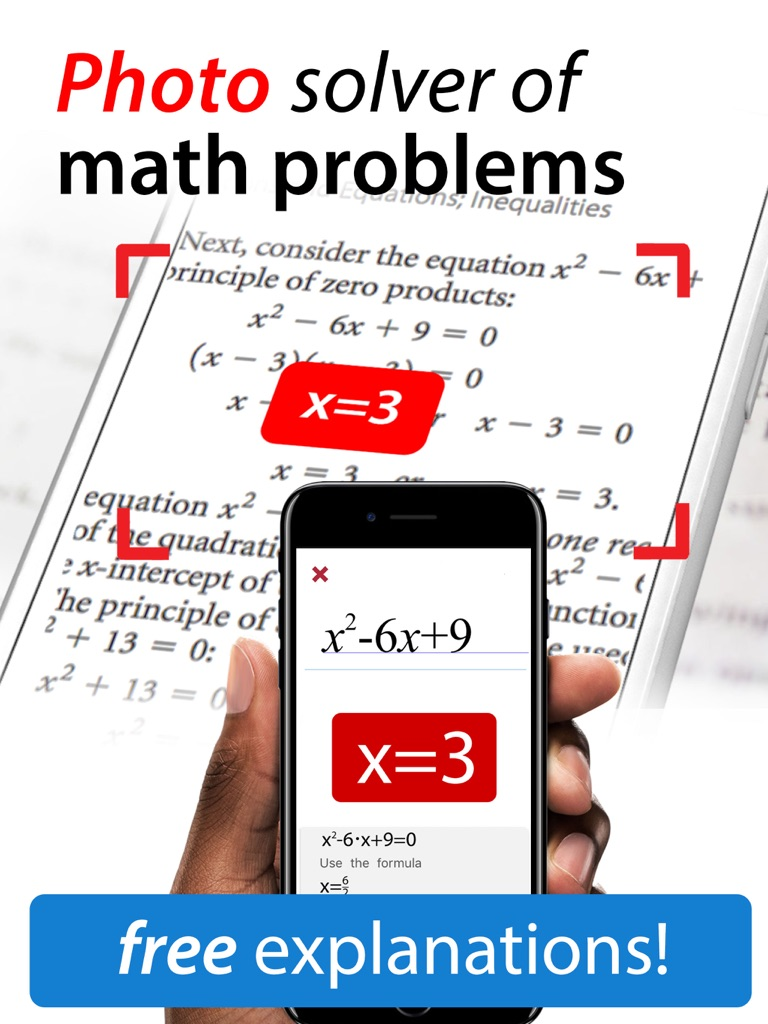# Math problem solver, photo – Sergey VladimirovichMath problem solver, photo Sergey Vladimirovich Genre: Education Release Date: March 5, 2016

mathapp solver app helps pupils and students study their homework easy and fast

• Graphical Curve Sketching (Derivation, roots, extremes, inflection points, poles, asymptotical functions, intersection points)
Equations of higher degree (numerical solutions, substitution)
• Exponential, logarithmic and trigonometric equations (Substitution)
• Absolute value and inequations (Case distinction)
• Polynomials (Roots, factors)
• Integrals (By parts, by substitution, partial fraction decomposition), definite and indefinite integral
• Curve Sketching (Derivation, roots, extremes, inflection points)
• Simplifications and transformations (Signs, combining, factorizing, binomial decomposition, …)
• Fractions (adding, reducing, multiplying, simplifying, GCD, LCM, …)
• Logarithms and trigonometric functions (rad/deg)
• Linear equations
• Fractional equations
• Systems of equations (linear and non-linear)
• Polynomial long division
• Derivation (quotients, chain, powers, roots, trigonometry, sums, exponents, products, …)

Optional MyMath Subscription:
– \$2.0 monthly plan and \$10.00 unlimited plan available
– Payment will be charged to iTunes Account at confirmation of purchase
– Subscription automatically renews unless auto-renew is turned off at least 24-hours before the end of the current period
– Account will be charged for renewal within 24-hours prior to the end of the current period, and identify the cost of the renewal
– Subscriptions may be managed by the user and auto-renewal may be turned off by going to the user's Account Settings after purchase
– No cancellation of the current subscription is allowed during active subscription period